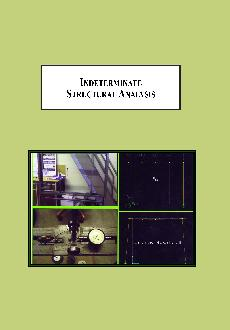# Indeterminate Structural AnalysisAuthor: Derucher, Kenneth N. Year: 2013 Pages: 128 ISBN: 0-7734-4470-X978-0-7734-4470-6 Price: \$139.95
This textbook covers the analysis of indeterminate structures by force method, displacement method and stiffness method in a total of six chapters which can be covered in a single course on indeterminate structural analysis. It includes an as-needed discussion of the unit load method, which is arguably the best method to calculate deflections when solving problems by the force method.

### Reviews

“Indeterminate Structural Analysis” is a must-have addition for advanced students and professionals that require knowledge at the tip of their fingers. An excellent resource.”
-Prof. James Scolaro,
California State University, Chico State

PREFACE
INTRODUCTION
CHAPTER 1 – ANALYSIS OF STATICALLY INDETERMINATE STRUCTURES BY THE FORCE METHOD

1.1 Basic Concepts of the Force method
1.1.1. List of Symbols and Abbreviations Used in the Force Method
1.2 Static Indeterminacy
1.3 Basic Concepts of the Unit Load Method for Deflection Calculation
1.4 Maxwell’s Theorem of Reciprocal Deflections
1.5 Application of Force method to Analysis of indeterminate Beams
1.5.1 Sign Convention
1.5.3. Structures with Several Redundant Forces 1.6 Application of the Force Method to Indeterminate Frames
1.7 Application of Force Method to Analysis of Indeterminate Trusses
1.8 Summary
Problems
CHAPTER 2 – DISPLACEMENT METHOD OF ANALYSIS: SLOPE-DEFLECTION METHOD

2.1 Basic Concepts of the Displacement Method
2.2 Basic procedure of the Slope-Deflection Method
2.2.1 Slope-Deflection Method
2.2.2 Sign Convention for Displacement Methods
2.2.3 Fixed-End Moments
2.3 Analysis of Continuous Beams by the Slope-Deflection Method
2.4 Analysis of Continuous Beams with Support Settlements by the Slope-Deflection Method
2.5 Application of the Slope-Deflection Method to Analysis of Frames Without Joint Movement
2.6 Derivation of Shear Condition for Frames (With Joint Movement)
2.7 Application of the Slope-Deflection Method to Analysis of Frames With Joint Movement
2.8 Summary
Problems
CHAPTER 3 – DISPLACEMENT METHOD OF ANALYSIS: MOMENT DISTRIBUTION METHOD

3.1 Basic Concepts of Moment Distribution Method
3.2 Stiffness Factor, Carry-over Factor and Distribution Factor
3.2.1 Stiffness Factor
3.2.2 Carry-Over Factor (CO)
3.2.3 Distribution Factor (DF)
3.3 Analysis of continuous Beams by Moment Distribution method
3.3.1 Basic Procedure for Moment Distribution
3.4 Analysis of a Continuous Beam with Support Settlement by Moment Distribution Method
3.5 Application of Moment Distribution to Analysis of Frames Without Sidesway
3.6 Application of Moment Distribution to Analysis of Frames with Sidesway
3.6.1 basic Concepts: Application of Moment Distribution to Analysis of Frames with Sidesway
3.7 Summary
Problems
CHAPTER 4 - DIRECT STIFFNESS METHOD: APPLICATION TO BEAMS

4.1 Basic Concepts of the Stiffness Method
4.2 Kinematic Indeterminacy
4.3 Relation Between Stiffness Method and Direct Stiffness Method
4.4 Derivation / Explanation of the Beam-Element Stiffness Matrix
4.4.1 Global/Structure Stiffness Matrix
4.5 Application of the Direct Stiffness Method to a Continuous Beam
4.6 Summary
Problems
CHAPTER 5 – DIRECT STIFFNESS METHOD: APPLICATION TO FRAMES

5.1 Derivation/Explanation of the Stiffness Matrix for a Frame Element
5.2 Application of the Direct Stiffness Method to a Frame
5.3 Summary
Problems
CHAPTER 6 – DIRECT STIFFNESS METHOD: APPLICATION TO TRUSSES

6.1 Derivation / Explanation of the Stiffness Matrix for a Truss Element
6.2 Application of the Direct Stiffness Method to a Truss
6.3 Summary
Problems
APPENDIX – A
REFERENCES